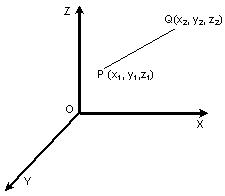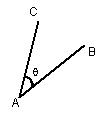Click to Chat

1800-1023-196

+91-120-4616500

CART 0

• 0

MY CART (5)

Use Coupon: CART20 and get 20% off on all online Study Material

ITEM
DETAILS
MRP
DISCOUNT
FINAL PRICE
Total Price: Rs.

There are no items in this cart.
Continue Shopping```Parallel Lines

Table of Content

Direction Cosines and Direction Ratios of a Line Joining two given Points

Angle Between Two Lines

Condition of Parallelism

Condition of Perpendicularity

We now Move on to Some Illustrations Based on the Concepts Discussed Above

Related ResourcesBefore we proceed towards the concept of parallel lines, we shall first discuss direction ratios and direction cosines of a line.

Direction Cosines and Direction Ratios of a Line Joining two given Points

The direction cosine of a line has the same meaning as the direction cosines of a vector. Any three numbers proportional to direction cosines of a line are called direction ratios. If we have two points say P(x1, y1, z1) and Q(x2, y2, z2), then the direction ratios of the line joining P and Q arex2 – x1 = a(say), y2 – y1 = b (say) and z2 – z1 = c (say).

Then direction cosines are

l = a/(√a2 + b2 + c2), m = b/(√a2 + b2 + c2), n = c/(√a2 + b2 + c2)

Angle Between Two Lines

Let θ be the angle between two straight lines AB and AC whose direction cosines are given by l1, m1, n1and l2, m2, n2 respectively. Then the angle between the lines AB and AC is given by cos θ = l1l2 + m1m2 + n1n2.Moreover, if the direction ratios of the two lines are a1, b1, c1and a2, b2, c2, then the angle between the two lines is given by

(a1a2+ b2b1+ c1c2) / √(a12 + b12 + c12) .√(a22 + b22 + c22)

The lines can either be parallel, coplanar or perpendicular. Whether they are parallel, coplanar or perpendicular can be decided with the help of their direction cosines.

You might like to view a related video

Condition of Parallelism

Since parallel lines have the same direction, it follows that the direction cosines of two or more parallel straight lines are the same. So in case of lines, which do not pass through the origin, we can draw a parallel line passing through the origin and direction cosines of that line can be found.

Hence, if we have two lines with dc’s (direction cosines) as l1, m1, n1 and l2, m2, n2 respectively then these two lines will be parallel if

l1/l2 = m1/m2 = n1/n2

We try to establish how the above result is obtained:

If the given lines are parallel, then q = 00 i.e. sin θ = 0

⇒ (l1m2 – l2m1)2 + (m1n2 – m2n1)2 + (n­­1l2 – n2l1)2

which is true, only when

l1m2 – l2m1 = 0, m1n2 – m2n1 = 0 and n­­1l2 – n2l1 = 0 ⇒ I1/l2 = m1/m2 = n1/n2

Similarly, a1/a2 = b1/b2 = c1/c2.

Condition of Perpendicularity

If the given lines are perpendicular, then θ = 900 i.e. cos θ = 0

⇒ l1l2 + m1m2 + n1n2 = 0 or a1a2 + b1b2 + c1c2 = 0.

We have, sin2θ = 1 – cos2θ

= (i12 + m12 + n12)(i22 + m22 + n22) – (l1l2 + m1m2 + n1n2)2

= (l1m2 – l2m1)2 + (m1n2 – m2n1)2 + (n1l2 – n2l1)2

⇒ sin θ = ± √Σ(l1m2 – l2m1)2 .

We now Move on to Some Illustrations Based on the Concepts Discussed Above

Illustration:

Find the direction cosines of two lines which are connected by the relations l–5m + 3n = 0 and 7l2 + 5m2 – 3n2 = 0.

Solution:

The given relations are

l – 5m + 3n = 0 ⇒ l = 5m – 3n   ……(1)

and 7l2 + 5m2 – 3n2 = 0               ……(2)

Putting the value of l from (1) in (2), we get

7(5m – 3n)2 + 5m2 – 3n2 = 0

or, 180m2 – 210mn + 60n2 = 0

or, (2m – n)(3m – 2n) = 0

∴ m/n = 1/2 or 2/3

when m/n = 1/2 i.e. n = 2m

∴ l = 5m – 3n = –m or 1/m = –1

Hence, we have l/–1 = m/1 = n/2 = √ (l2 + m2 + n2) / √ {(–1)2 + l2 + 22} = 1/√6

So, direction cosines of one line are –1/√6, 1/√6, 2/√6

Again when m/n = 2/3 or n = 3m/2

∴ l = 5m – 3.3m/2 = m/2 or 1/m = 1/2

Thus, m/n = 2/3 and l/m = 1/2 giving l/1 = m/2 = n/3 = 1/√12 + 22 + 32 = 1/√14

∴ The direction cosines of the other line are 1/√14, 2/√14, 3/√14.

Illustration:

Find the direction ratios and direction cosines of the line joining the points A (6, –7, –1) and B(2, –3, 1).

Solution:

Direction ratios of AB are (6-2, –7-(-3), –1-1)

(4, – 4, – 2) = (2, – 2, – 1)

Hence, we have a = 2, b = -2, c = -1. So, a2 + b2 + c2 = 9.

Direction cosines are (± 2/3, ± 2/3, ± 1/3).

Illustration:

Show that two lines having direction ratios –1, 3, 2 and 2, 2, –2 are perpendicular.

Solution:

We know that the liens are perpendicular if a1a2 + b1b2 + c1c2 = 0.

Hence we compute a1a2 + b1b2 + c1c2. We have

a1 = –1, b1 = 3, c1 = 2 and a2 = 2, b2 = 2, c2 = –2

a1a2 + b1b2 + c1c2 = (–1)(2) + (3)(2) + (2)(–2) = –2 + 6 – 4 = 0

This shows that the lines are perpendicular.

Illustration:

(a) Find the equation of the plane passing through the points (2, 1, 0), (5, 0, 1) and (4, 1, 1).

(b) If P is the point (2, 1, 6), then the point Q such that PQ is perpendicular to the plane given above and the mid-point of PQ lies on it. (2003)

Solution:

(a) The equation of the plane passing through (2, 1, 0) is

a(x-2) + b(y-1) + c(z-0) = 0

It also passes through (5, 0, 1) and (4, 1, 1).

Hence, we have 3a – b + c = 0

and 2a + 0b + c = 0

On solving the above equations we get,

a/-1 = b/ -1 = c/2

Hence, the required equation of plane is

-(x-2) – (y-1) + 2(z-0) = 0

- x + 2 – y + 1 + 2z = 0

x + y – 2z = 3.

(b) Let the coordinate of Q be (α, β, γ).

The equation of line PQ is given by

(x-2)/1 = (y-1)/1 = (z-6)/-2

Now, the mid-point of P and Q is ((α+2)/2, (β+1)/2, (γ+6)/2), which lies in a line PQ.

[(α+2)/2 – 2] / 1 = [(β+1)/2 – 1] / 1 = [(γ+6)/2 – 6]/ -2

Simplifying this we get the value as 2.

Hence, α = 6, β = 5 and γ = -2.

Hence, the coordinates of point Q are Q(6, 5, -2).

Related Resources

Look into the past year papers to get an idea about the types of questions asked in the exam.

Various Recommended Books of Mathematics are just a click away.

To read more, Buy study materials of 3D Geometry comprising study notes, revision notes, video lectures, previous year solved questions etc. Also browse for more study materials on Mathematics here.
```### Course Features

• 731 Video Lectures
• Revision Notes
• Previous Year Papers
• Mind Map
• Study Planner
• NCERT Solutions
• Discussion Forum
• Test paper with Video Solution